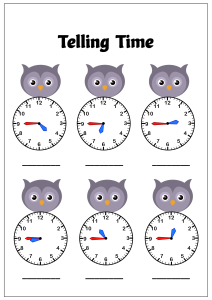# Math Worksheet: Telling Time to the Quarter Hour (Quarter To) - Owl Theme Time

Practice telling time on analog clocks to the quarter hour (Quarter To) - Help kids learn telling time to the Quarter Hour using the analog clocks. These worksheets will help kids learn and practice telling and writing the time correct to the quarter hour. Introduce the concept of time using our Tell time to hour or half hour or quarter hour Owl theme worksheets collection.Viewed by 3285 parents and educators in last 932 days
Age Group
Subjects

#### Related Worksheets

Related worksheet for Kids

• Math

• Math

• Math

• Math

• Math

• Math